# Lesson 27 Solving Linear Trigonometric Equations IB Math

• Slides: 32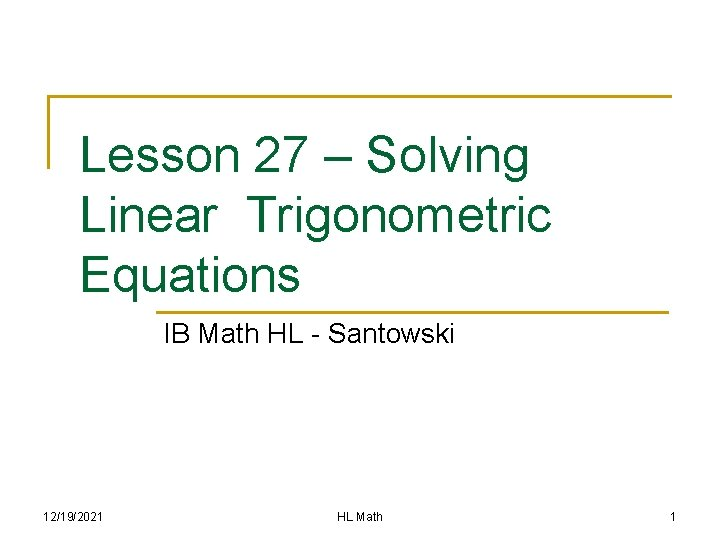Lesson 27 – Solving Linear Trigonometric Equations IB Math HL - Santowski 12/19/2021 HL Math 1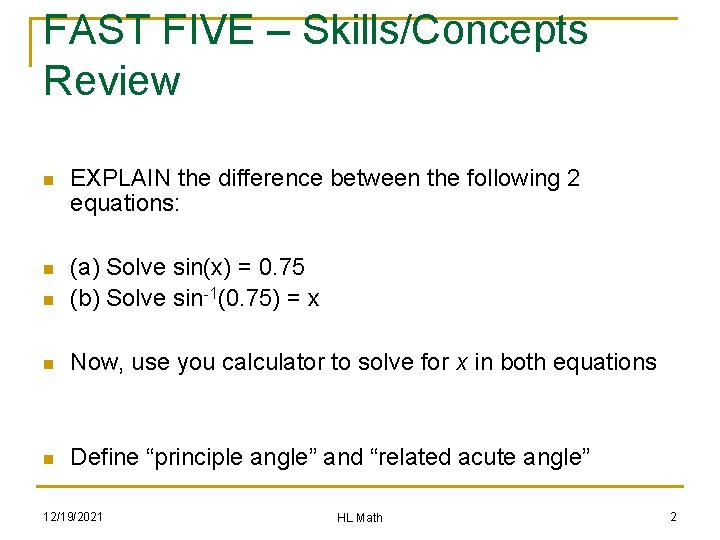FAST FIVE – Skills/Concepts Review n EXPLAIN the difference between the following 2 equations: n n (a) Solve sin(x) = 0. 75 (b) Solve sin-1(0. 75) = x n Now, use you calculator to solve for x in both equations n Define “principle angle” and “related acute angle” 12/19/2021 HL Math 2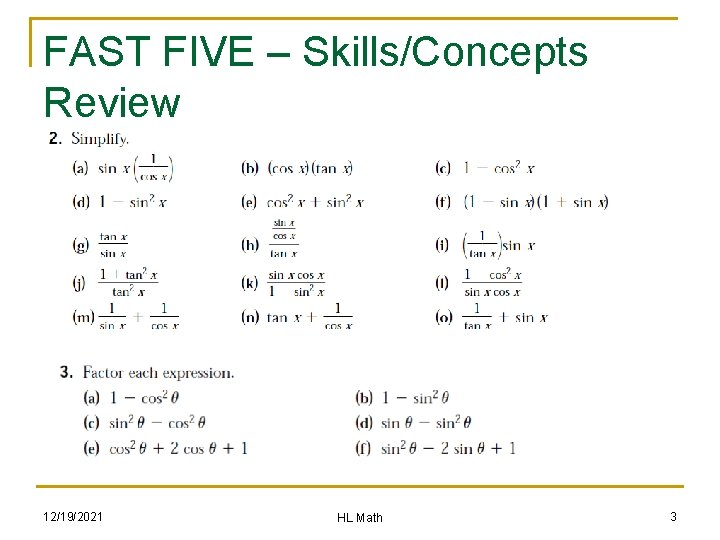FAST FIVE – Skills/Concepts Review 12/19/2021 HL Math 3(A) Review n n We have two key triangles to work in two key ways (i) given a key angle, we can determine the appropriate value of the trig ratio & (ii) given a key ratio, we can determine the value(s) of the angle(s) that correspond to that ratio We know what the graphs of the two parent functions look like and the 5 key points on each curve 12/19/2021 HL Math 4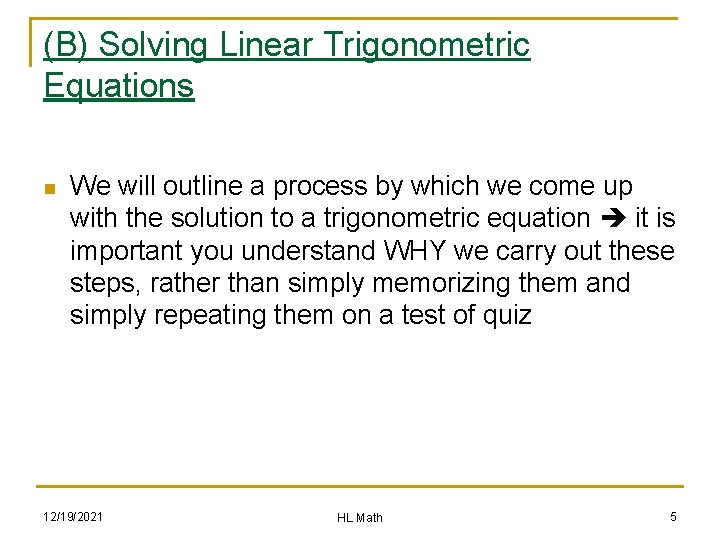(B) Solving Linear Trigonometric Equations n We will outline a process by which we come up with the solution to a trigonometric equation it is important you understand WHY we carry out these steps, rather than simply memorizing them and simply repeating them on a test of quiz 12/19/2021 HL Math 5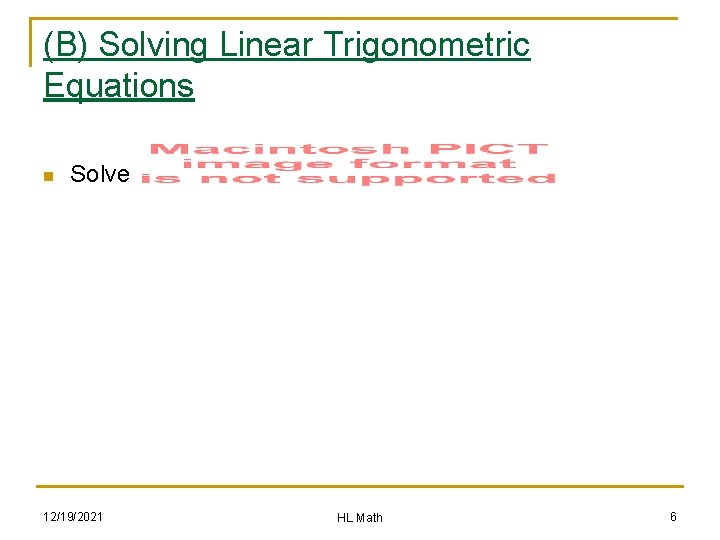(B) Solving Linear Trigonometric Equations n Solve 12/19/2021 HL Math 6(B) Solving Linear Trigonometric Equations n Work with the example of sin(θ) = -√ 3/2 n Step 1: determine the related acute angle (RAA) from your knowledge of the two triangles Step 2: consider the sign on the ratio (-ve in this case) and so therefore decide in what quadrant(s) the angle must lie Step 3: draw a diagram showing the related acute in the appropriate quadrants Step 4: from the diagram, determine the principle angles n n n 12/19/2021 HL Math 7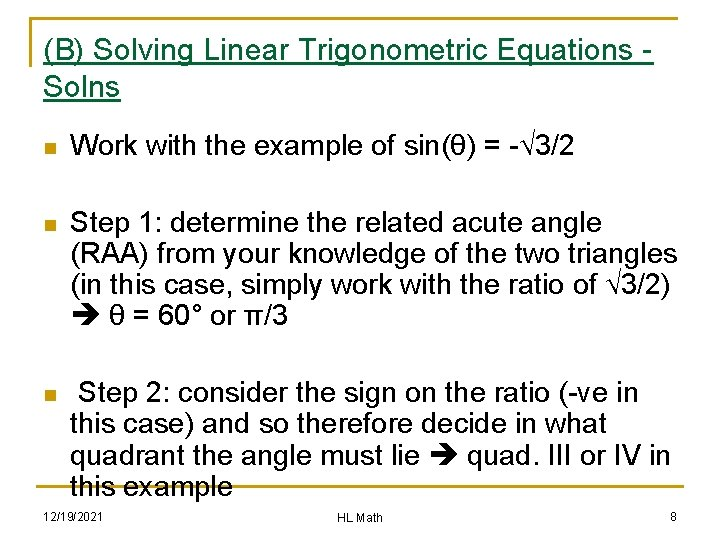(B) Solving Linear Trigonometric Equations Solns n Work with the example of sin(θ) = -√ 3/2 n Step 1: determine the related acute angle (RAA) from your knowledge of the two triangles (in this case, simply work with the ratio of √ 3/2) θ = 60° or π/3 n Step 2: consider the sign on the ratio (-ve in this case) and so therefore decide in what quadrant the angle must lie quad. III or IV in this example 12/19/2021 HL Math 8(B) Solving Linear Trigonometric Equations n Step 3: draw a diagram showing the related acute in the appropriate quadrants n Step 4: from the diagram determine the principle angles 240° and 300° or 4π/3 and 5π/3 rad. 12/19/2021 HL Math 9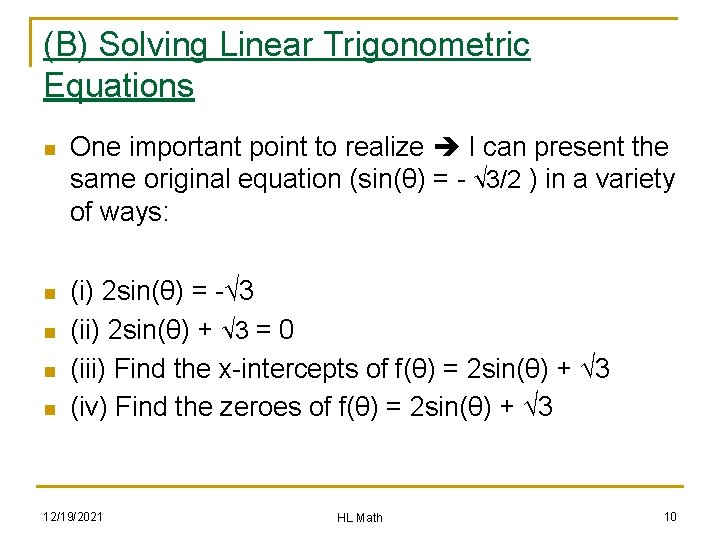(B) Solving Linear Trigonometric Equations n n n One important point to realize I can present the same original equation (sin(θ) = - √ 3/2 ) in a variety of ways: (i) 2 sin(θ) = -√ 3 (ii) 2 sin(θ) + √ 3 = 0 (iii) Find the x-intercepts of f(θ) = 2 sin(θ) + √ 3 (iv) Find the zeroes of f(θ) = 2 sin(θ) + √ 3 12/19/2021 HL Math 10(B) Solving Linear Trigonometric Equations n A second important point to realize I can ask you to solve the equation in a variety of restricted domains (as well as an infinite domain) n (i) Solve 2 sin(θ) = -√ 3 on [-2π, 2π] (ii) Solve 2 sin(θ) + √ 3 = 0 on [-4π, π/2) (iii) Find the x-intercepts of f(θ) = 2 sin(θ) + √ 3 on (2π, 0) (iv) Find ALL the zeroes of f(θ) = 2 sin(θ) + √ 3, given an infinite domain. n n n 12/19/2021 HL Math 11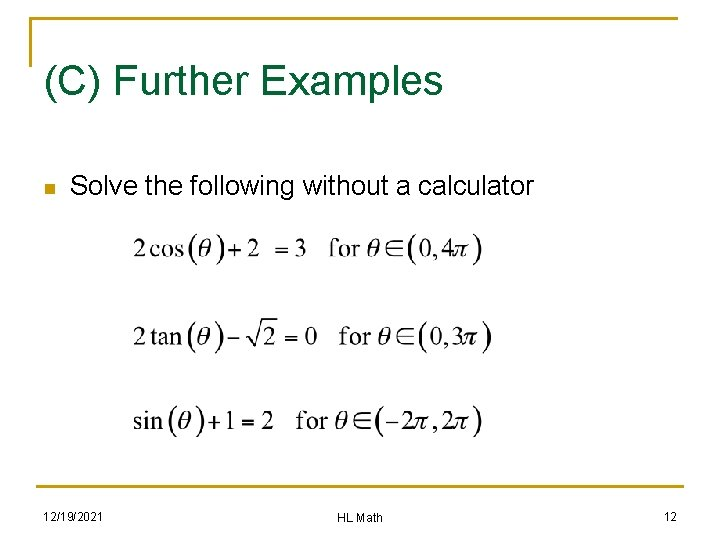(C) Further Examples n Solve the following without a calculator 12/19/2021 HL Math 12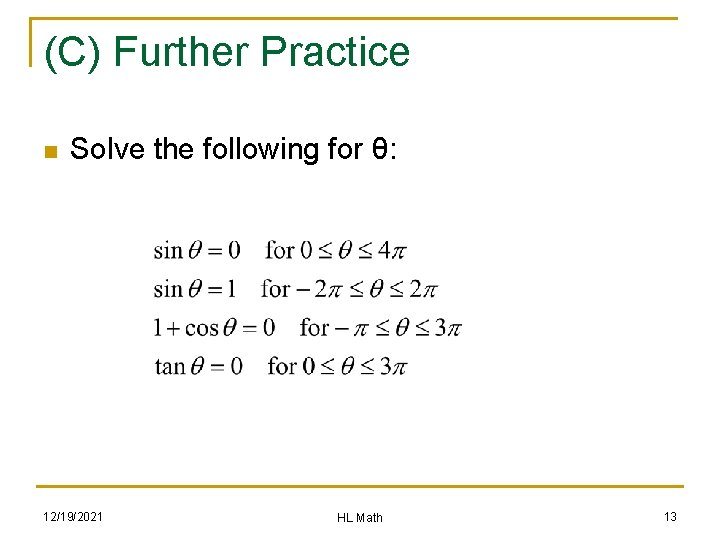(C) Further Practice n Solve the following for θ: 12/19/2021 HL Math 13(C) Further Practice n Solve without a calculator 12/19/2021 HL Math 14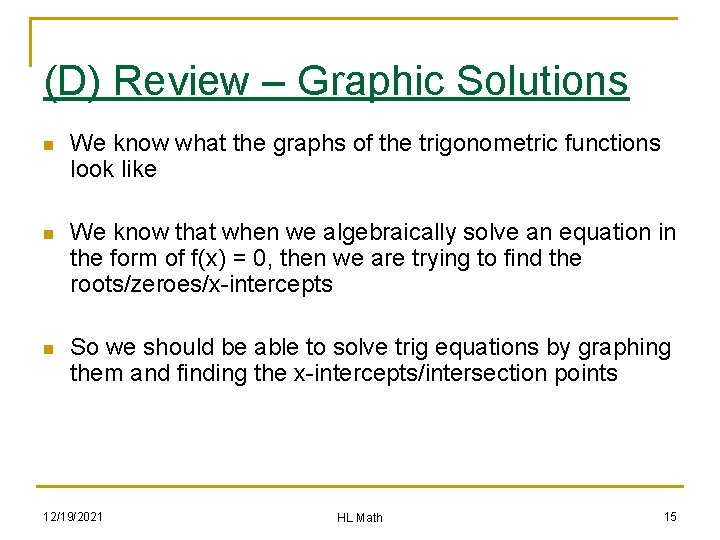(D) Review – Graphic Solutions n We know what the graphs of the trigonometric functions look like n We know that when we algebraically solve an equation in the form of f(x) = 0, then we are trying to find the roots/zeroes/x-intercepts n So we should be able to solve trig equations by graphing them and finding the x-intercepts/intersection points 12/19/2021 HL Math 15(E) Examples (with Technology) n Solve the equation 3 sin(x) – 2 = 0 12/19/2021 HL Math 16(E) Examples n Solve the equation 3 sin(x) – 2 = 0 n The algebraic solution would be as follows: n We can set it up as sin(x) = 2/3 so x = sin-1(2/3) giving us 41. 8° (and the second angle being 180° - 41. 8° = 138. 2° Note that the ratio 2/3 is not one of our standard ratios corresponding to our “standard” angles (30, 45, 60), so we would use a calculator to actually find the related acute angle of 41. 8° n 12/19/2021 HL Math 17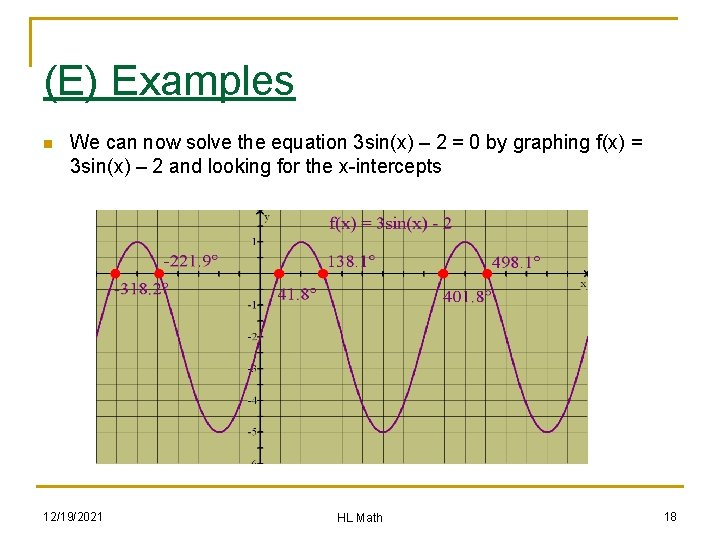(E) Examples n We can now solve the equation 3 sin(x) – 2 = 0 by graphing f(x) = 3 sin(x) – 2 and looking for the x-intercepts 12/19/2021 HL Math 18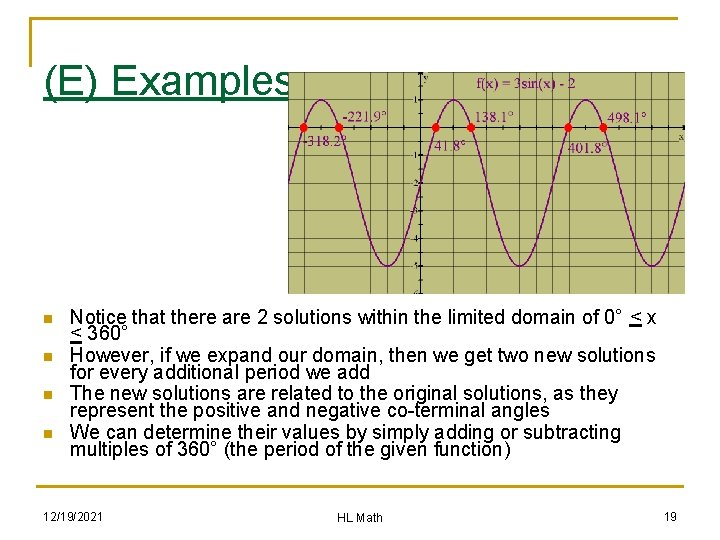(E) Examples n n Notice that there are 2 solutions within the limited domain of 0° < x < 360° However, if we expand our domain, then we get two new solutions for every additional period we add The new solutions are related to the original solutions, as they represent the positive and negative co-terminal angles We can determine their values by simply adding or subtracting multiples of 360° (the period of the given function) 12/19/2021 HL Math 19(E) Examples n Solve the following equations: 12/19/2021 HL Math 20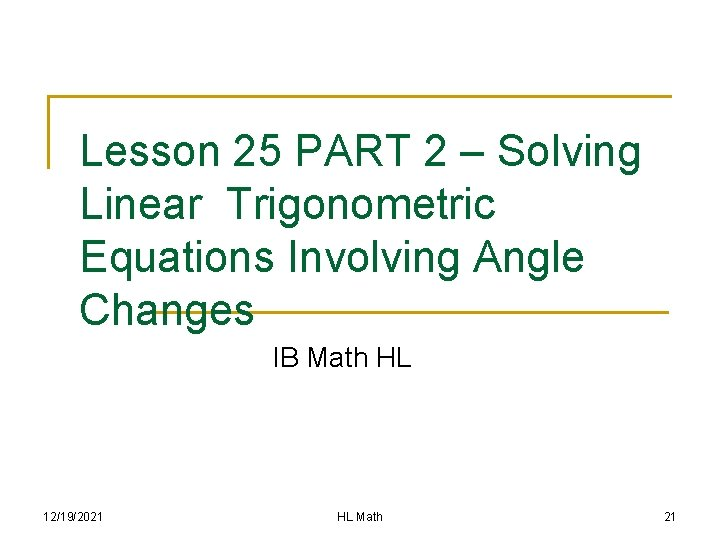Lesson 25 PART 2 – Solving Linear Trigonometric Equations Involving Angle Changes IB Math HL 12/19/2021 HL Math 21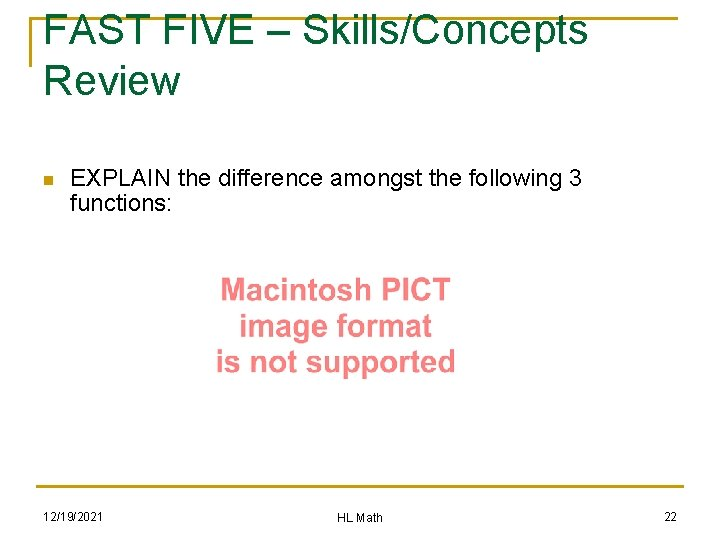FAST FIVE – Skills/Concepts Review n EXPLAIN the difference amongst the following 3 functions: 12/19/2021 HL Math 22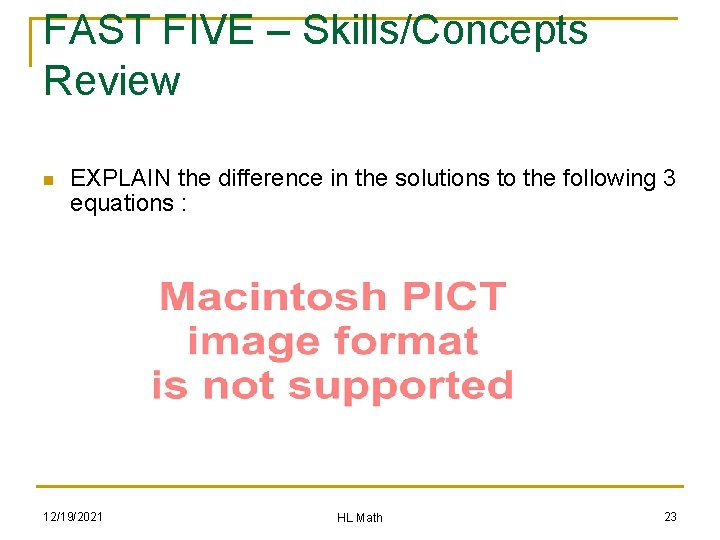FAST FIVE – Skills/Concepts Review n EXPLAIN the difference in the solutions to the following 3 equations : 12/19/2021 HL Math 23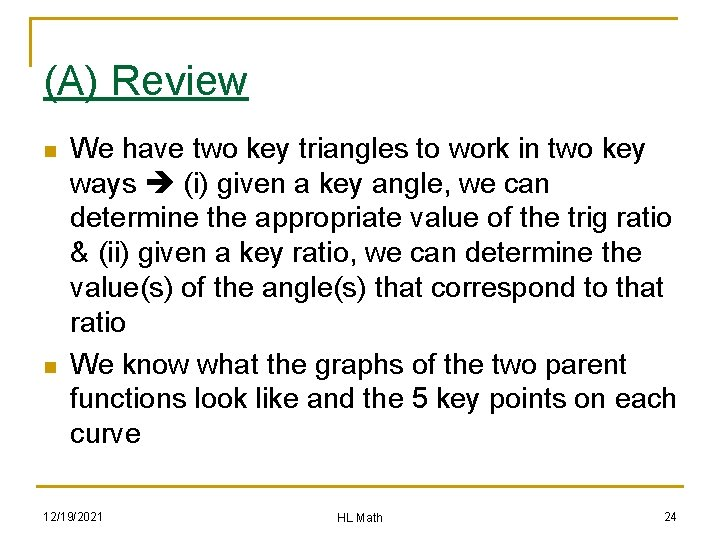(A) Review n n We have two key triangles to work in two key ways (i) given a key angle, we can determine the appropriate value of the trig ratio & (ii) given a key ratio, we can determine the value(s) of the angle(s) that correspond to that ratio We know what the graphs of the two parent functions look like and the 5 key points on each curve 12/19/2021 HL Math 24(B) Example Set #1 n Without the use of a calculator, prepare an algebraic solution to the following equations: 12/19/2021 HL Math 25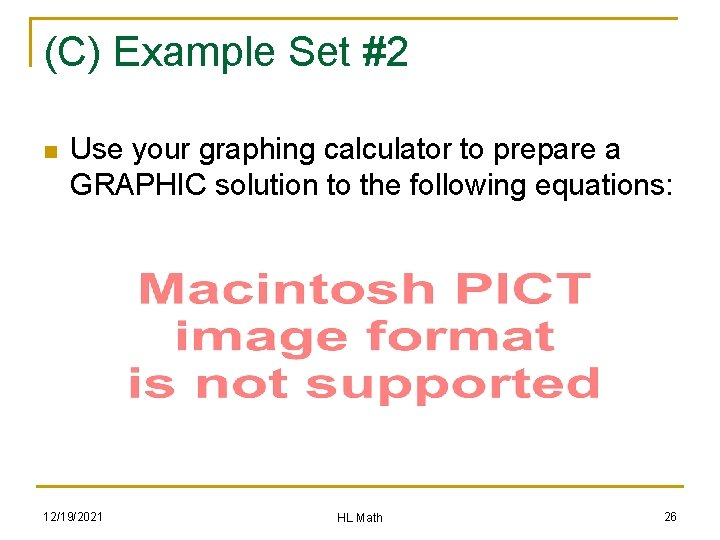(C) Example Set #2 n Use your graphing calculator to prepare a GRAPHIC solution to the following equations: 12/19/2021 HL Math 26(D) Example Set #3 n Without the use of a calculator, prepare an algebraic solution to the following equations: 12/19/2021 HL Math 27(D) Example Set #4 n Without the use of a calculator, prepare an algebraic solution to the following equations: 12/19/2021 HL Math 28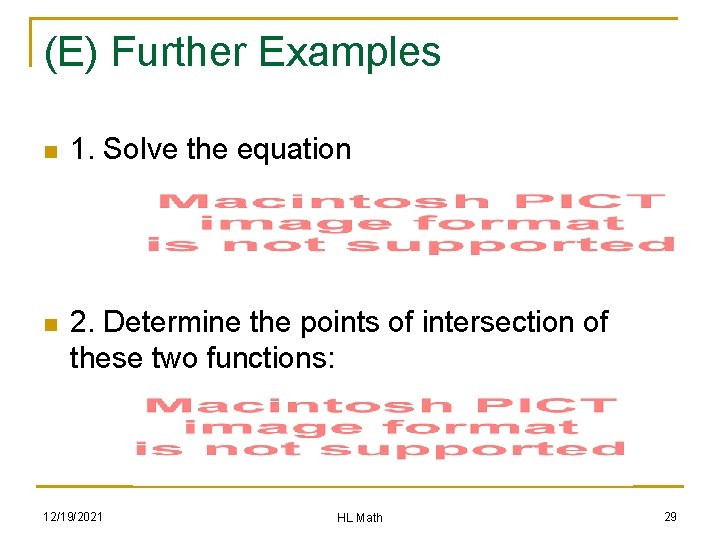(E) Further Examples n 1. Solve the equation n 2. Determine the points of intersection of these two functions: 12/19/2021 HL Math 29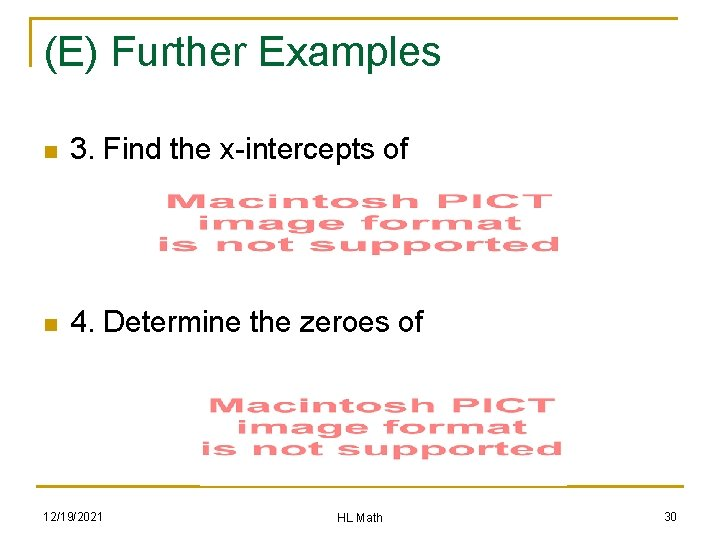(E) Further Examples n 3. Find the x-intercepts of n 4. Determine the zeroes of 12/19/2021 HL Math 30(E) Further Examples n 5. An equation that produces the solutions of x = 150° + 360°n, nεI as well as 330° + 360°n, nεI is ……. 12/19/2021 HL Math 31(F) Solving Equations with Technology n The monthly sales of lawn equipment can be modelled by the following function, where S is the monthly sales in thousands of units and t is the time in months, t = 1 corresponds to January. n (a) How many units will be sold in August? (b) In which month will 70 000 units be sold? (c) According to this model, how many times will the company sell 70 000 units over the next ten years? n n 12/19/2021 HL Math 32